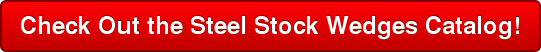## Steel Manufacturing Blog: Keeping it Steel

The steel wedge is an extremely important aspect of any construction process.  Another way to understand the power of the steel wedge is by understanding the structural bolt.  At first, it might seem as though that the two products have nothing in common, but in reality they are the same thing.

Both the wedge and the screw are simple machines derived from the principle of the incline.  The fundamental theory is that it takes less energy to move an object up along a slope than it does to lift it straight from above.  While the steel wedge operates in a straight line, the structural bolt does the same thing, only in a spiral line.  Both are still fundamentally considered wedges, or “inclines,” depending on your preferred term.

Calculating the power whether expansive, or “lifting” (Wedge), or compressive (Structural Bolt) the equation is the same. Length of incline divided by distance of lift.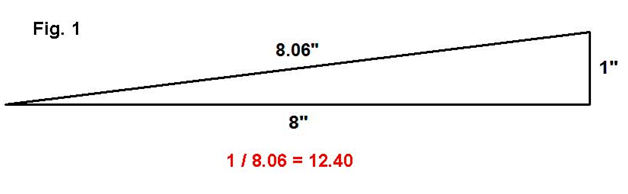In the case of Figure 1, pictured above, the lifting force of 12.40 can be viewed as a percent.  To lift an object straight up, it requires a force equal to 100 percent of the weight of the object.  To lift the same object using a steel wedge, requires only 12.40 percent of that force.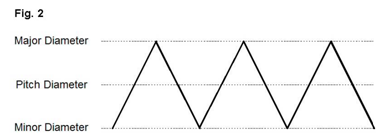Relating the same equation to the structural bolt, the incline remains the same.  It is measured as the distance between two threads.  The length is now measured as the circumference of the bolt barrel.  Note, Figure 2, above, shows this can be measured from the outer edge of the thread, the inner, or at the mid-point.  In addition, all three diameters are smaller than the actual diameter.  That is, a 1” diameter bolt has a major diameter of .9980”, a pitch diameter of .9134” and a minor diameter of .8446”.  To keep this explanation simple, we used 1” as the diameter.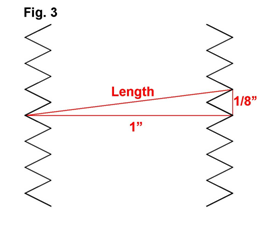The circumference of a circle with a 1” diameter is 3.14”. Using UNC bolt specifications, there are 8 threads per inch in a 1” diameter bolt, so each thread rises a distance of 1/8”.  Figure 3, above, illustrates how the bolt is actually a wedge.  With this, the new variable is the length. However, because the length is the circumference, it now measures 3.14”.

1/8 ÷ 3.14 = 0.0398

At this time, it should be recalled that the 0.0398 result can actually be viewed as a percent, and for simplicity’s sake the number can be rounded up to an even 4 percent.  That means that only 4 percent of the pressure is required to produce 100 percent of the force.

Until now, only the wedge action of the bolt has been mentioned.  The leverage created by the handle of the wrench tightening the bolt only compounds this pressure.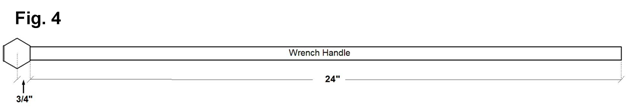In Figure 4, above, we see the standard hex head for a 1” bolt is 1-1/2” from flat to flat. With the pivot point being the center or axis, it is 3/4” to any flat edge.  The 24” handle then has a ratio of 1:32.  So the percentage shown above of 4 percent, or 25 times advantage, is now multiplied again by 32. The full advantage being created by this wedge and lever is 800 times the actual pressure being applied.  With these results, it’s easy to see how the incline for a steel wedge or structural bolt makes everything easier.

To see the specific kinds of steel wedges we offer, click below!